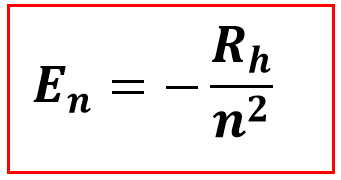# Problem: (a) How much energy is required to ionize hydrogen when it is in the ground state?eV(b) How much energy is required to ionize hydrogen when it is in the state for which n = 5?eV

###### FREE Expert Solution

The problems requires us to calculate for the energy required to ionize Hydrogen at different states.

a. We want to get the energy required to ionize hydrogen when it is in the ground state, hence the formula to use is the Bohr and Balmer equation.Where:

En= Energy at a state of n

Rh= Rydberg Constant (2.18x10-18 J)

= energy level

98% (258 ratings)###### Problem Details

(a) How much energy is required to ionize hydrogen when it is in the ground state?
eV

(b) How much energy is required to ionize hydrogen when it is in the state for which n = 5?
eV

Frequently Asked Questions

What scientific concept do you need to know in order to solve this problem?

Our tutors have indicated that to solve this problem you will need to apply the Bohr Model concept. You can view video lessons to learn Bohr Model. Or if you need more Bohr Model practice, you can also practice Bohr Model practice problems.